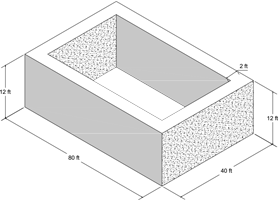### Math Notes

Subjects

#### Solid Geometry Solutions

##### Topics || Problems

How many cubic yards of material are needed for the foundation of a barn 40 ft by 80 ft, if the foundation is 2 ft thick and 12 ft high?$$Vol_{p}= L ~ x ~ W ~ H$$

$$Vol = Vol_{outside} - Vol_{inside}$$

$$Vol_{outside}= 40x80x12$$

$$Vol_{outside}= 38400 ~ ft^3$$

To calculate the volume of the inside rectangular parallelepiped, solve first the dimensions.

The length is $$80-4 = 76 ~ft$$ and the width is $$40-4 = 36 ~ft$$

$$Vol_{inside}= 36x76x12$$

$$Vol_{inside}= 32832 ~ft^3$$

$$Vol = 38400 - 32832$$

$$Vol = 5568 ~ft^3$$

$$Vol = \frac{5568}{27} ~yd^3$$, $$1 yd^3 = 27 ft^3$$

$$Vol = 206.22 ~yd^3$$